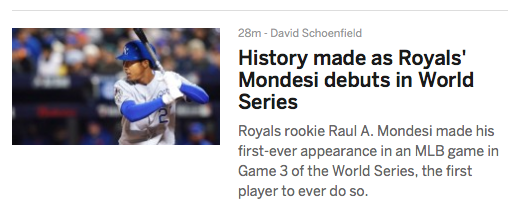## Friday, October 30, 2015

### 44 59 79 244 422 | ESPN World Series Game 3 Headlines, and Raul Mondesi Recognition

I noticed my World Series video has been at this view count for at least an hour, without moving up, which is hard to believe on the day of Game 3 of the World Series, with the internet and all.  I recall that after my May 12 prediction of a possible Subway Series, my video was frozen at 188 views for a week.  This was creepy, because later the same day of the prediction, train #188 would crash in Philadelphia, on the way to New York.

After 188 views, it jumped up to 422 views, then froze at that same number, 422 for months.

Here it is again.

Strane enough, ESPN has been using the reflection of that number all night.

Mets are currently up 3-9, in New York.

New = 5+5+5 = 15
York = 7+6+9+2 = 24
New York = 39

And let us not forget this is the 111th World Series.

New = 14+5+23 = 42 (NYC = 14+25+3 = 42)
York = 15+25+18+11 = 69
New York = 111 (111th World Series)The Royals won the World Series in '85.  The start date connects to the possible end date of the 2015 World Series.

10/19/85 = 10+19+85 = 114
TBD, Game 7 of 2015 World Series would be on 11/4, November 4

ANYHOW, here is something else that stands out to me.  Look at this article on the cover of ESPN, right now, with the headline above.  It is about former MLB-Star, Raul Mondesi.

Raul = 9+1+3+3 = 16
Mondesi = 4+6+5+4+5+1+9 = 34/43
Raul Mondesi = 50/59

Raul = 18+1+21+12 = 52
Mondesi = 13+15+14+4+5+19+9 = 79
Raul Mondesi = 131

3/12/1971 = 3+12+19+71 = 105
3/12/1971 = 3+12+(1+9+7+1) = 33
3/12/1971 = 3+1+2+1+9+7+1 = 24
3/12/71 = 3+12+71 = 86

Notice that Raul Mondesi is currently 44-years old, and his life numbers and name Gematria make '59' and '79'.

All of these numbers are conceding.44-years oldMurder = 79 = Mondesi (From Gematria above) Murder = 13+21+18+4+5+18 = 79 Raul Mondesi better watch his ass.

1.2.